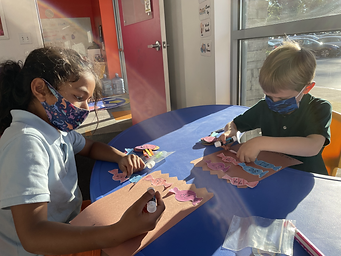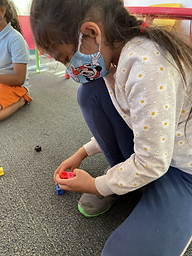## Ms. Melissa

### Target 1​

###### Lesson Type:

Continuation

Algebra

:

Sequence

Use arithmetic to define a sequence and calculate the next unit in a series.

###### 1:

Study a given arithmetic sequence to determine what rule explains the sequence (i.e., 2-4-6-8-10…each number is the sequence gets bigger by 2).

###### 2:

Write an arithmetic rule to explain a given sequence.

###### 3:

Understand that a pattern can be described by stating the rule that it is following.

1st

###### Vocabulary:

Number Pattern, Sequence, Subtract

Activities:

Students rolled dice to come up with a two-digit starting number. Students wrote this on lollipop heads. Students then rolled one dye to come up with a number to subtract and made sequences on the other lollipops accordingly.

Students found the missing numbers in sequences written on candy cut-outs.

Students used lollipops and candy cut-outs to create goodie bags.### Home Exploration

###### Guiding Questions:## Absent Students:

### Target 2

:

###### 1:

Understand that the same number of blocks can be arranged in numerous ways (setting the foundation that different figures can have the same volume even if they look different).

2nd

###### Vocabulary:

Volume

Activities:

Students used Unifix cubes to represent different volumes.### Home Exploration

###### Guiding Questions:### Target 3

:

###### Vocabulary:

Activities:### Home Exploration